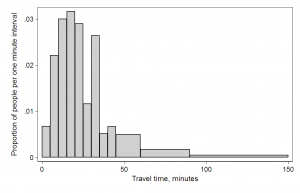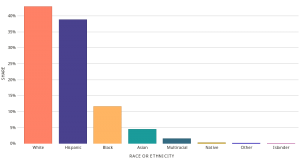# What is the difference between a BarChart and a Histogram ?

A Histogram represents the distribution of a numerical variable.  A bar-chart is typically used to compare numeric values corresponding to categorical variables.

To construct a histogram:

• X-axis: Usually the range of values is binned. In other words, the entire range is divided into a series of intervals and each interval occupies a slot on the X axis.
• Y-axis: The number of times a value occurred in each interval is shown on the Y axis.

Example: Histogram of number of people who arrived at various time intervalsTo construct a Vertical Barchart :

X axis: Categorical Labels

Y-axis : Numeric values corresponding to the categorical variable

Example: Comparing the percentage of population of various ethnicities